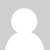### 5v power protection from AC transformer

Currently evaluating the voltage stepdown circuit and can't figure out what keeps the 5v Arduino power protected.

Dividing the AC down to 1vpp, wouldn't that still push through the resistor causing the 5v power to fluctuate?

I am in the US and plan on having two voltage sensing circuits, and two CT circuits.  All this powering off the 5v arduino power with no diodes, confuses me. It seems that the AC from the transformers + the AC from the CTs would all effect each other by screwing up the voltage dividers.

Any help would be awesome.

Thanks!!### Re: 5v power protection from AC transformer

Moving along it looks like impedance with a little capacitor help smooth things out for the 5v rail.

I am experimenting with the arduino digital outputs for the 5v source, giving each ct and ac circuit an output set high. This isolates the rail using the arduino clamps.

So far so good. Hoping for thoughts ....

### Re: 5v power protection from AC transformer

hello, apologies for slow reply. I've just spent some time simulating this in LTspice and it is interesting with the resistor magnitudes used here, an internal resistance of 0.2 Ohms (typical AA battery at 20C is 0.1Ohms I think) for both power supplies and the ADC input impedence of 100MOhms there is no visible fluctuation on the 5V line.

Without the 10uf capacitor there is a 3.6mV peak-to-peak fluctuation on the 2.5V voltage divider if the voltage divider is made up of 2x 470k resistors or 0.08mV when both are 10k.

If you then add in the 10uf capacitor the voltage divider fluctuation goes down to 0.005mV peak-to-peak for both the 470k and 10k combination.

So well outside the range of significance.

If the components where different, if the voltage divider for the AC voltage input was much smaller and the internal resistance of the power supply much larger say >50Ohms and the ADC input impedence was much smaller then we would see some issues.

Just to make sure we are talking about the same circuit, your reffering to the mains AC v3 circuit right?